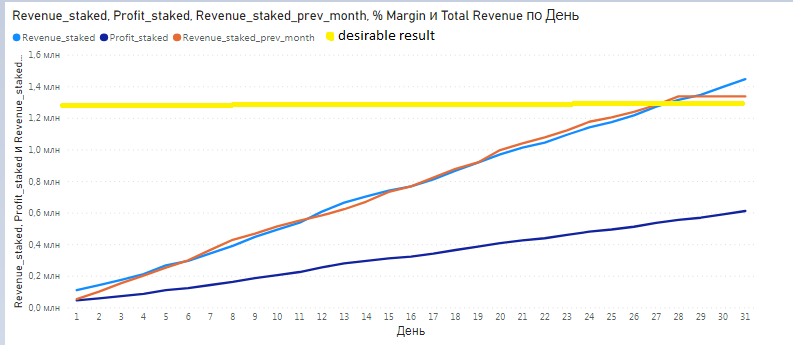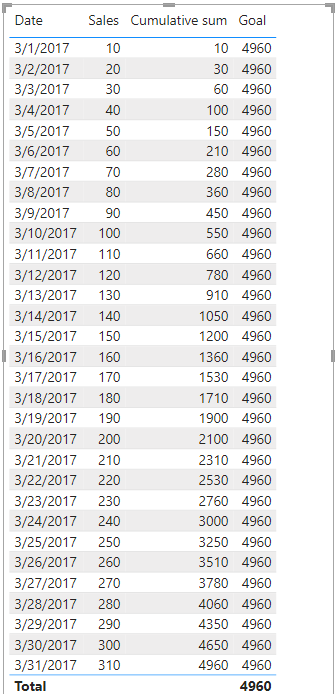cancel
Showing results for
Did you mean:Helper I

## Recurring monthly total every day for a month

Hello! I want to make a calculated measure in DAX so it calculates total sales per month and repeat that number for every day of the month on the graph. I am allowed to achieve calculate staked sales from day by day, but can't understand how to calculate total sales for month and repeat that number every day (yellow line). The formulas for staked sales look like this:

Revenue_staked = CALCULATE(SUMX(AW_Sales, AW_Sales[OrderQuantity] * RELATED(AW_Product_Lookup[ProductPrice])),DATESMTD(AW_Calendar_Lookup[Date]))

Profit_staked = CALCULATE(SUMX(AW_Sales, AW_Sales[OrderQuantity] * RELATED(AW_Product_Lookup[ProductPrice])),DATESMTD(AW_Calendar_Lookup[Date])) - CALCULATE(SUMX(AW_Sales, AW_Sales[OrderQuantity] * RELATED(AW_Product_Lookup[ProductCost])),DATESMTD(AW_Calendar_Lookup[Date]))1 ACCEPTED SOLUTIONCommunity Support

Hi, @bombom

I understand your question, you can check out the following methods.
Measure:

``Cumulative sum = CALCULATE(SUM('Table'[Sales]),FILTER(ALL('Table'),[Date]<=SELECTEDVALUE('Table'[Date])))``
``Goal = CALCULATE(SUM('Table'[Sales]),FILTER(ALL('Table'),MONTH([Date])=3&&YEAR([Date])=2017))``Result:Is this the result you expect?

Best Regards,

Community Support Team _Charlotte

If this post helps, then please consider Accept it as the solution to help the other members find it more quickly.

4 REPLIES 4Community Support

Hi, @bombom

Can you simplify your data by providing some sample data tests? Sensitive information can be removed in advance. What kind of expected results do you expect?

Best Regards,

Community Support Team _Charlotte

If this post helps, then please consider Accept it as the solution to help the other members find it more quickly.Helper I

Hello, @v-zhangti

Thank you for reaching my question.

The goal is to sum total revenue of the month and duplicate this number across each day of the month, so it will create a one-way flat line chart.

For example, on the screenshot is a chart from dataset, March 2017, that calculates revenue inside one month day by day accumulatively. On the last day the revenue equals 1 448 594,08. In other words - it is a total revenue of the month. The goal is to take this total revenue, 1 448 594,08, and duplicate it for everyday of the month.Community Support

Hi, @bombom

I understand your question, you can check out the following methods.
Measure:

``Cumulative sum = CALCULATE(SUM('Table'[Sales]),FILTER(ALL('Table'),[Date]<=SELECTEDVALUE('Table'[Date])))``
``Goal = CALCULATE(SUM('Table'[Sales]),FILTER(ALL('Table'),MONTH([Date])=3&&YEAR([Date])=2017))``Result:Is this the result you expect?

Best Regards,

Community Support Team _Charlotte

If this post helps, then please consider Accept it as the solution to help the other members find it more quickly.Helper I

Hello @v-zhangti

Thank you so much, looks good!